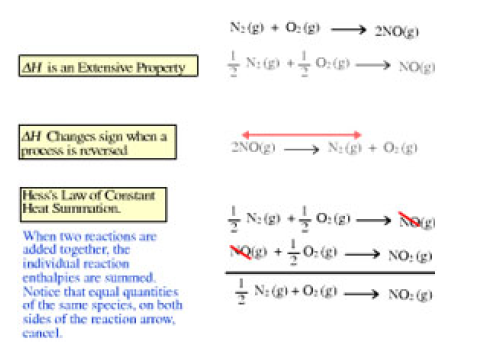# Problem: Click on the ΔH changes sign when a process is reversed button within the activity and analyze the relationship between the two reactions that are displayed. The reaction that was on the screen when you started and its derivative demonstrate that the reaction enthalpy, ΔH, changes sign when a process is reversed.Consider the reactionB(s) + 3/2 O2(g) → B2O3(s), ΔH = − 1270kJWhat will ΔH be for the reaction if it is reversed?Express your answer with appropriate units.Reaction 1: C3H8(g) + 5 O2(g) → 3 CO2(g) + 4 H2O(g), ΔH1 = − 2043 kJReaction 2: 3C3H8(g) + 15 O2(g) → 9 CO2(g) + 12 H2O(g), ΔH2 = ?Express your answer to four significant figures and include the appropriate units.Open the activity depicting Hess's Law.

###### FREE Expert Solution
99% (252 ratings)View Complete Written Solution
###### Problem Details

Click on the ΔH changes sign when a process is reversed button within the activity and analyze the relationship between the two reactions that are displayed. The reaction that was on the screen when you started and its derivative demonstrate that the reaction enthalpy, ΔH, changes sign when a process is reversed.

Consider the reaction
B(s) + 3/2 O2(g) → B2O3(s), ΔH = − 1270kJ

What will ΔH be for the reaction if it is reversed?

Reaction 1: C3H8(g) + 5 O2(g) → 3 CO2(g) + 4 H2O(g), ΔH= − 2043 kJ
Reaction 2: 3C3H8(g) + 15 O2(g) → 9 CO2(g) + 12 H2O(g), ΔH2 = ?

Express your answer to four significant figures and include the appropriate units.

Open the activity depicting Hess's Law.